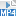## Content

### Expanding

#### Brackets

Collections of terms may be grouped together in algebra using brackets. Such a collection may then be multiplied by another such term or collection of terms. To simplify such an expression may involve expanding the brackets using the distributive law.

#### Example

Simplify

$2x^2y(3x-5y).$

#### Solution

$$2x^2y(3x-5y)=6x^3y-10x^2y^2$$.

Note that we used the index laws.

#### Binomials

We can also expand pairs of brackets. To expand the expression $$(a+b)(x+y)$$, we must multiply every term in the first bracket by every term in the second. Hence,

\begin{align*} (a+b)(x+y) &=a(x+y)+b(x+y)\\ &=ax+ay+bx+by. \end{align*}

Expressions such as this, involving a pair of brackets each containing two terms, are sometimes referred to as binomials. With practice, the middle step above can be left out.

#### Example

Expand and simplify

$(2x+3)(5x-2).$

#### Solution

\begin{align*} (2x+3)(5x-2)&=2x(5x-2)+3(5x-2)\\ &=10x^2-4x+15x-6\\ &=10x^2+11x-6. \end{align*}

Note that the first expansion can be left out.

The process can be extended to brackets containing more than two terms.

#### Example

Expand and simplify

$(x-2)(x^2+2x+4).$

#### Solution

\begin{align*} (x-2)(x^2+2x+4)&=x(x^2+2x+4)-2(x^2+2x+4)\\ &=x^3+2x^2+4x-2x^2-4x-8\\ &=x^3-8. \end{align*}
##### Exercise 2

Expand and simplify

$(x-1)(x^{n-1}+x^{n-2}+ \dots +x^2+x+1).$

Screencast of exercise 2#### Special expansions

There are three special binomial products that arise frequently and their patterns should be learned off by heart, rather than expanding them step by step as we did above.

\begin{align*} (x+y)^2 &= x^2+2xy+y^2\\ (x-y)^2 &= x^2-2xy+y^2\\ (x+y)(x-y) &= x^2-y^2 \end{align*}

The first two of these identities are often referred to as perfect squares, while the third is called the difference of squares.

#### Example

Expand

1. $$(x-2)(x+2)$$
2. $$(x+5)^2$$
3. $$(2x-3)^2$$.

#### Solution

1. $$(x-2)(x+2) =x^2-4$$
2. $$(x+5)^2= x^2+10x+25$$
3. $$(2x-3)^2= 4x^2 -12x+9$$.
##### Exercise 3

By expanding both sides (separately) prove that

$(a+b+c)(ab+bc+ca)-abc = (a+b)(b+c)(c+a).$

Next page - Content - Factoring